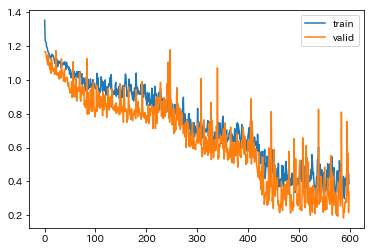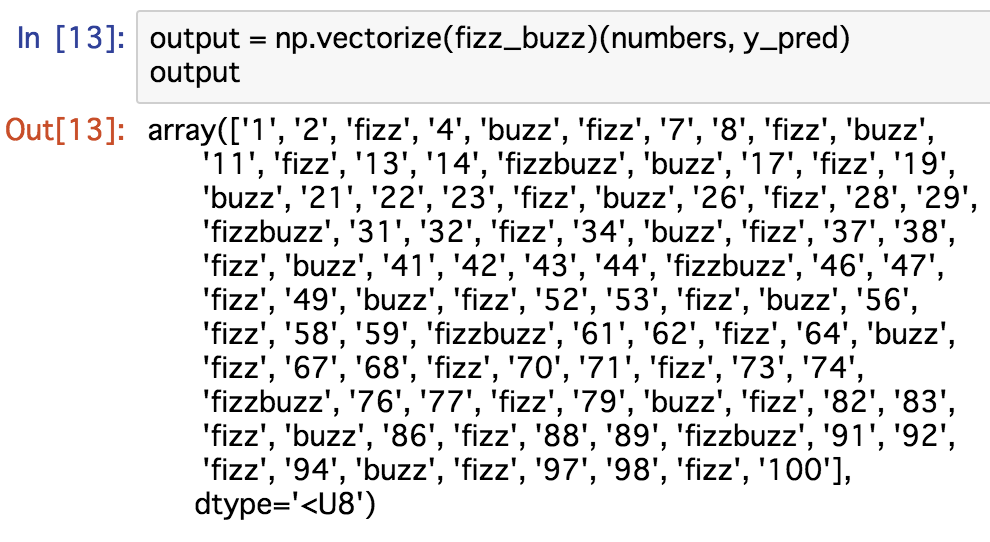u++の備忘録

# Fizz Buzz in PyTorch

In this article, I utilize MLP(Multilayer perceptron) by PyTorch to solve Fizz Buzz problem.

This challenge is highly inspired by the following page, and I use the same situation.

You can see the Japanese version here:

### Train data & Test data

• Train data: FizzBuzz data of N = 101~10000
• Test data: FizzBuzz data of N = 1~100

### Format of X, y

X are binary encoded N.

```def binary_encode(i, num_digits):
return np.array([i >> d & 1 for d in range(num_digits)])
```
```for i in range(1, 10):
print(binary_encode(i, 10))
```
```[1 0 0 0 0 0 0 0 0 0]
[0 1 0 0 0 0 0 0 0 0]
[1 1 0 0 0 0 0 0 0 0]
[0 0 1 0 0 0 0 0 0 0]
[1 0 1 0 0 0 0 0 0 0]
[0 1 1 0 0 0 0 0 0 0]
[1 1 1 0 0 0 0 0 0 0]
[0 0 0 1 0 0 0 0 0 0]
[1 0 0 1 0 0 0 0 0 0]```

y are {0, 1, 2, 3}. it means that this is a classification task for 4 values.

```def fizz_buzz_encode(i):
if   i % 15 == 0: return 3
elif i % 5  == 0: return 2
elif i % 3  == 0: return 1
else: return 0

def fizz_buzz(i, prediction):
return [str(i), "fizz", "buzz", "fizzbuzz"][prediction]
```

These X and y are used for training.

### Prepare for Valid data

#### library

```import torch
from torch import nn, optim
from torch.utils.data import (Dataset,
TensorDataset)
import numpy as np
import matplotlib.pyplot as plt
plt.rcParams['font.family'] = 'IPAPGothic'
%matplotlib inline

torch.manual_seed(1)    # reproducible
from fastprogress import master_bar, progress_bar
```

#### Depart Valid data

Train data are consist of 923 data, and first 100 are departed as Valid data.

```NUM_DIGITS = 10
NUM_HIDDEN = 100
BATCH_SIZE = 128
X = np.array([binary_encode(i, NUM_DIGITS) for i in range(101, 2 ** NUM_DIGITS)])
y = np.array([fizz_buzz_encode(i) for i in range(101, 2 ** NUM_DIGITS)])

X_train = X[100:]
y_train = y[100:]
X_valid = X[:100]
y_valid = y[:100]
```

### Convert to torch

```X_train = torch.tensor(X_train, dtype=torch.float32)
X_valid = torch.tensor(X_valid, dtype=torch.float32)
y_train = torch.tensor(y_train, dtype=torch.int64)
y_valid = torch.tensor(y_valid, dtype=torch.int64)
```

### Model definition

```net = nn.Sequential(
nn.Linear(10, 100),
nn.ReLU(),
nn.BatchNorm1d(100),
nn.Linear(100, 4),
)

loss_fn = nn.CrossEntropyLoss()
optimizer = optim.Adam(net.parameters(), lr = 0.05)

ds = TensorDataset(X_train, y_train)
```

### Training

```train_losses = []
test_losses = []

for _ in progress_bar(range(600)):
running_loss = 0.0

net.train()

for i, (xx, yy) in enumerate(loader):
y_pred = net(xx)
loss = loss_fn(y_pred, yy)

loss.backward()
optimizer.step()
running_loss += loss.item()

train_losses.append(running_loss / i)

net.eval()
y_pred = net(X_valid)
test_loss = loss_fn(y_pred, y_valid)
test_losses.append(test_loss.item())
```### Apply to Test data

```numbers = np.arange(1, 101)
X_test = np.array([binary_encode(i, NUM_DIGITS) for i in range(1, 101)])
X_test = torch.tensor(X_test, dtype=torch.float32)

net.eval()
_, y_pred = torch.max(net(X_test), 1)

output = np.vectorize(fizz_buzz)(numbers, y_pred)
print(output)
```Now, we can get "successful" result. Accuracy is 95%.

```from sklearn.metrics import accuracy_score

y_true = np.array([fizz_buzz_encode(i) for i in range(1, 101)])
print(accuracy_score(y_true, y_pred))
```
`0.94999999999999996`

The implementation can be seen in my GitHub: